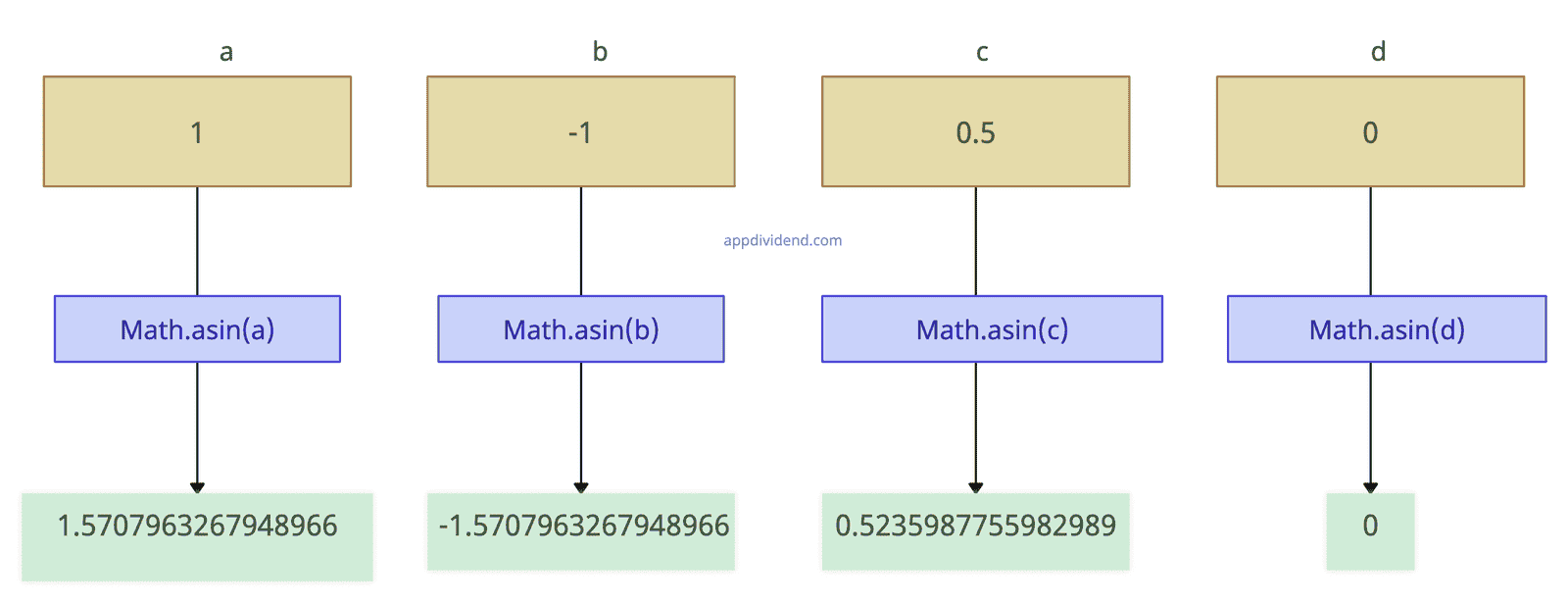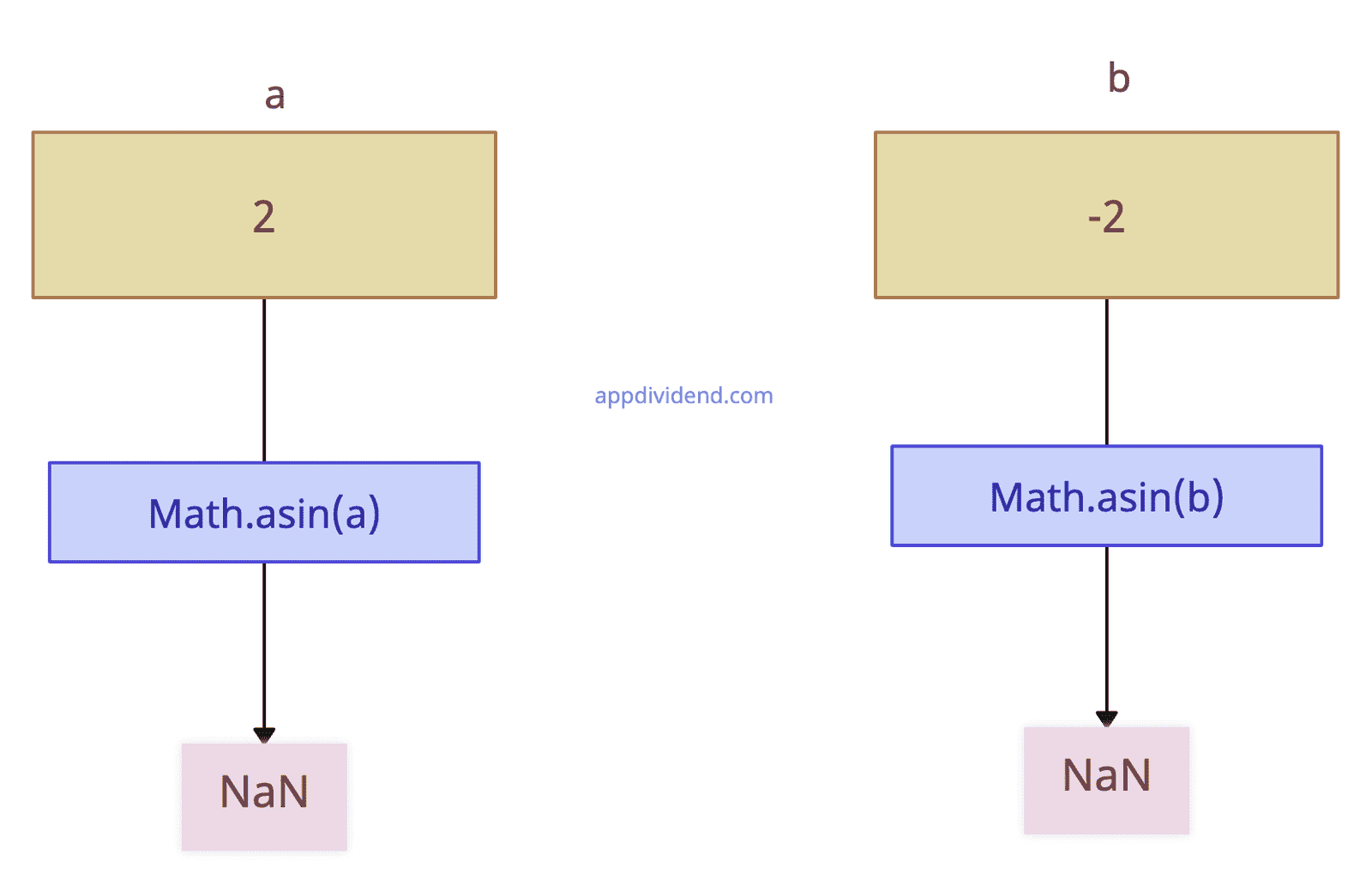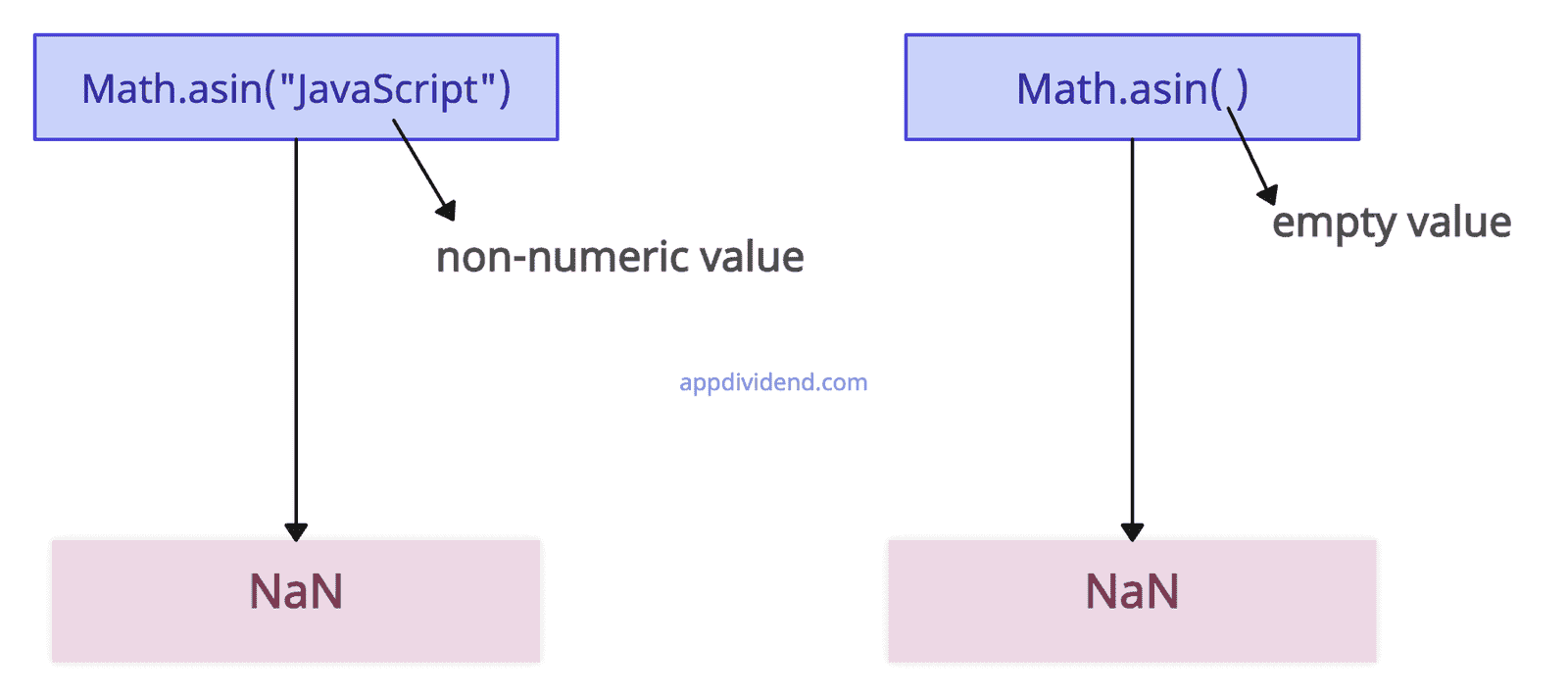# JavaScript Math asin() Function

JavaScript Math asin() method is used to calculate the arcsine(in radians) of a number of a number.

## Syntax

``Math.asin(x) ``

## Parameters

x(required): It is the number for which you want to calculate the arcsine. The number should be in the range of -1 to 1.

## Return Value

Returns a numeric value between -pi/2 and pi/2 radians.

If the argument is either non-numeric or greater than 1, or less than -1, it returns NaN.

## Visual RepresentationExample 1: How to Use Math asin() Function

``````var a = 1;   //positive number
var b = -1;  //negative number
var c = 0.5; //floating number
var d = 0;

console.log(Math.asin(a));
console.log(Math.asin(b));
console.log(Math.asin(c));
console.log(Math.asin(d));``````

Output

``````1.5707963267948966
-1.5707963267948966
0.5235987755982989
0``````

## Example 2:Handling Value Outside the -1 to 1 Range``````var a = 2;
var b = -2;

console.log(Math.asin(a));
console.log(Math.asin(b));``````

Output

``````NaN
NaN``````

## Example 3:Handling non-numeric and empty value``````console.log(Math.asin("JavaScript")); //non-numeric value
console.log(Math.asin()); //empty value
``````

Output

``````NaN
NaN``````

## Browser Compatibility

1. Google Chrome 1 and above
2. Edge 12 and above
3. Firefox 1 and above
4. Opera 3 and above
5. Safari 1 and above

This site uses Akismet to reduce spam. Learn how your comment data is processed.